# Degree of Total Leverage

A strategy that involves borrowing money to invest in any financial instrument or business.

Leverage is a strategy that involves borrowing money to invest in any financial instrument or business. This leads to a rise in the volatility of the company's earnings.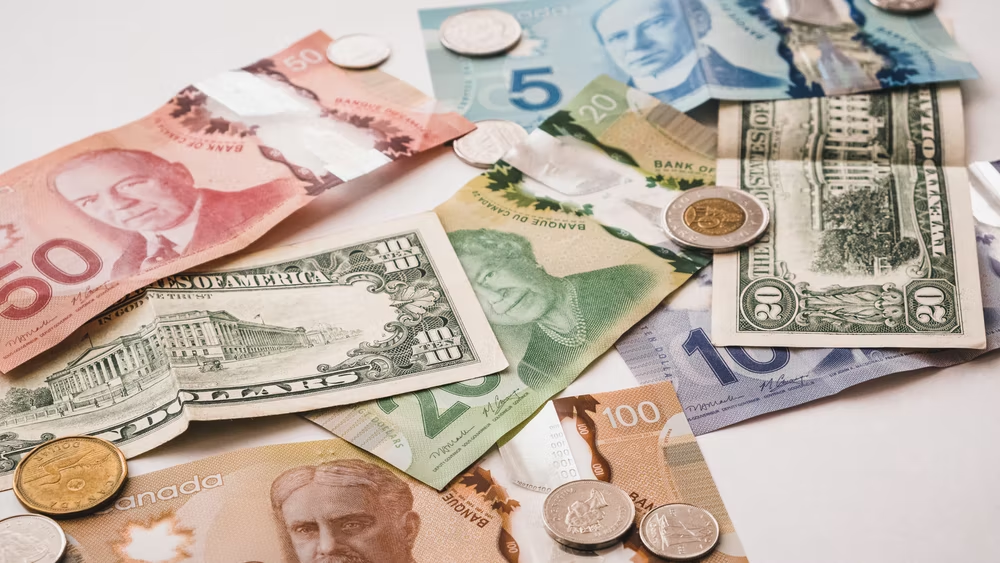Hence, the higher the degree, the higher the risk associated with that company's stock. Therefore, during the valuation of such a company, a higher discount rate is used.

A business's cost structure impacts the risk related to future earnings and cash flows. A company's cost structure is made up of a variety of variable and fixed expenses. The level of production and sales determines the degree of variable costs.

The price of products acquired for resale, the price of acquiring new equipment, and the cost of materials or supplies, shipping and delivery fees, hourly worker pay, sales commissions, and production or sales bonuses.

NOTE

Fixed costs remain the same regardless of the company's production and revenue.

What are the different ways in which it can be used?

It is a tool used by businesses to launch new initiatives, fund the purchase of inventories, and grow their operations.

Borrowing money can be more advantageous for many organizations than using equity or selling assets to fund transactions.

There is no requirement to give up ownership. However, it can benefit the firm when a business employs leverage, such as by issuing bonds, instead of bringing on new investors or issuing more stock.

It can be beneficial for small enterprises and startups that do not have a lot of funding or assets.2. In Personal Finance:

It is the term for borrowing money to buy something or possibly grow your money. Here are the following ways it can be used for personal finance:

• Education Loan
• Car loan
• Home Loan
• Personal Loan

3. In Investing:

Using it in investments can give investors a solid tool to boost their returns, but it also carries some significant risks. Buying on margin is another term for leverage in investing.

It is a strategy that carries a significant risk of loss and should be used cautiously, especially by new investors.

Professional traders and investors use it considerably to use their capital more effectively.

It increases a professional's ability to choose where to invest their capital. With leverage, they can potentially invest in many companies at once with fewer quantities of capital and more debt, thus increasing their purchasing power (and related returns).

Any business's degree can be divided into two parts - Operating Leverage (OL) and Financial Leverage (FL).

## Operating Leverage (OL)

OL assesses how much a company or project can raise operating income by increasing revenue. A company with significant OL creates sales with a high gross margin and low variable costs.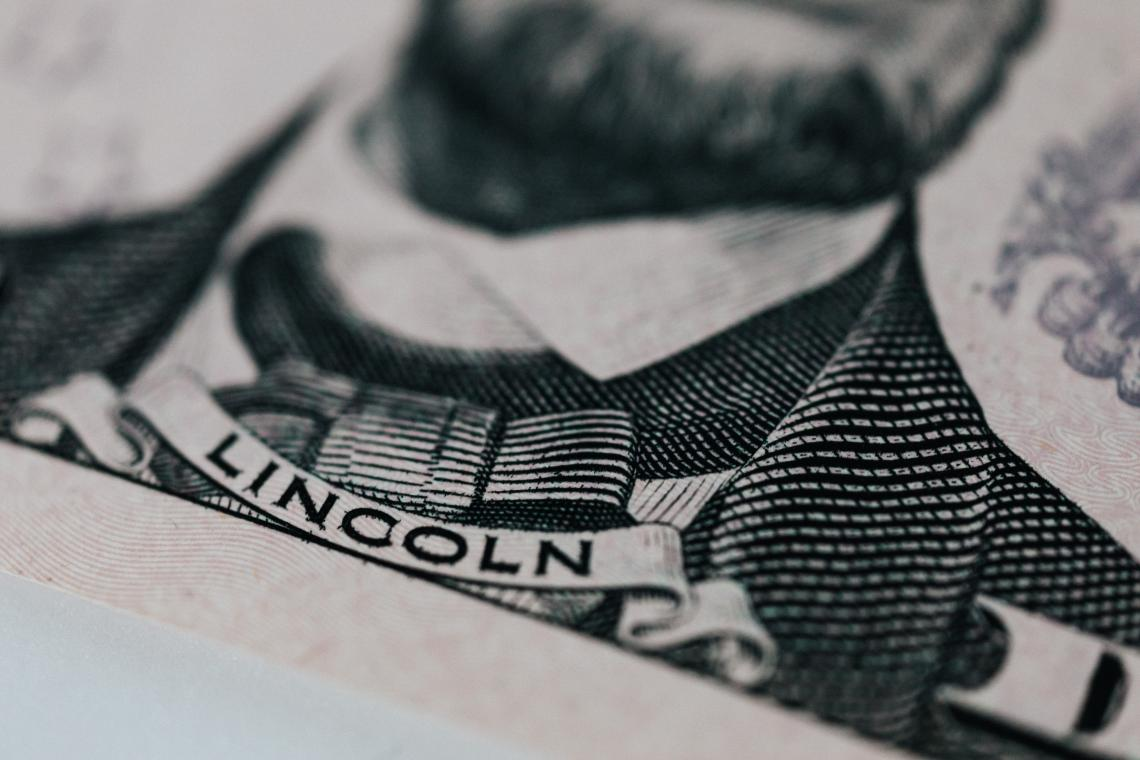Companies that have high fixed costs tend to have higher turnover-sensitive net income. In other words, if the number of products sold by the company changes, its net income will change drastically.

High OL suggests that the company can try to extract more revenue from each additional sale.

NOTE

When a company's cost structure is primarily comprised of fixed costs, it is not needed to incur additional expenses whenever a new client or larger order from an existing client arises.

The company doesn't need to invest much money to meet the increasing demand because it already has essential assets, such as

• manufacturing facilities,
• equipment, and
• those highly paid executives.

But it also has a downside; whether the business is booming or not, the company needs to pay up the fixed cost.

On the other hand, businesses with minimal OL have variable cost structures, which puts them in a better position to thrive in difficult economic times.

When the economy is in a downturn, variable expenses drop, making businesses more volatile in response to changing conditions. This can help a company make money even when it generates less income.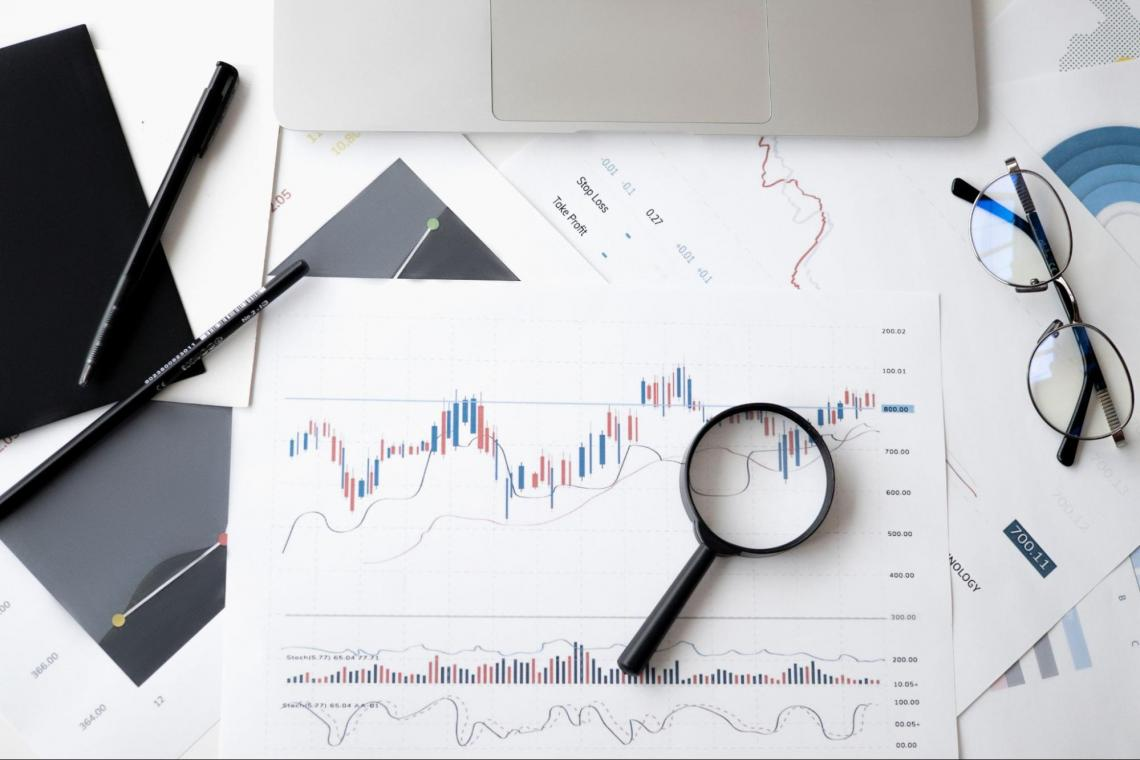These cost reductions aid in increasing the profit margin, and earnings grow more quickly than sales. This calculates the breakeven point for selling prices to cover all the costs.

Operating Income elasticity is known as the Degree of OL. That means we will try to find variations in operating income and the number of products/units sold. Therefore, the higher the ratio, the higher the OL.

How do we calculate the degree of OL?

Degree of OL = Contribution Margin (Total sales - Variable costs)/ Earnings before interest and taxes

Or

DOL = (Percentage change in Operating Income)/ (Percentage change in units sold)

Where,

Operating income = number of units sold * (Price per unit - Variable cost per unit) - Fixed Operating Costs

Industries that invest upfront in manufacturing a product yet spend relatively little on creating and distributing it tend to have substantial OL. This applies to both software developers and pharmaceutical businesses.

Alternatively, merchants, distributors, wholesalers, retailers, etc., have little OL since the cost of products sold is largely a variable cost.

What is Operating risk? It can be more challenging for a company to adjust its operational costs to changes in sales if the fixed costs are high (relative to variable costs).

The proportion of fixed and variable costs varies significantly depending on the type of business. The risk arising from fixed and variable expenses is known as operating risk. The concept of Elasticity is the connecting link between operational risk and the degree of OL.We will look at Elasticity in the next section.

## Elasticity

What is Elasticity? How does it affect risk factors?

Elasticity is a term used in economics to describe how much one economic variable changes in reaction to a change in another; if a good's price elasticity of demand is negative two, a ten percent price rise results in a twenty percent decrease in demand.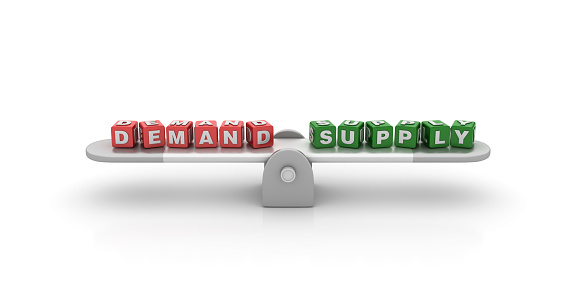Elasticity can be of various types.

1. Price Elasticity of Demand - Price elasticity of demand is the variance in demand in response to a change in price.

The ratio of the percentage change in the amount required to the percentage change in the price of a specific commodity may also be used to define it.

2. Price Elasticity of Supply - In economics, a metric known as price elasticity of supply (PES or Es) is used to demonstrate how responsive or elastic the quantity of an item or service is to changes in its price or cost.

3. Income Elasticity of Demand - The quantity of a good that is demanded in response to a change in consumer income is referred to as the income elasticity of demand in economics.

The ratio of the percentage change in the quantity required to the percentage change in income is used to calculate it.

4. Cross-Price Elasticity of Demand - Cross Price Elasticity of Demand (XED) assesses how two products are related to one another when the price of one varies. In other words, it determines how the change in the price of one product influences the demand for another.

5. The Elasticity of Scale - The percentage change in output caused by a total percent change in the utilization of all inputs is measured as the Elasticity of scale or output elasticity.

Using this concept, we can determine the risk, the income sensitivity to the number of units sold, or how much a business needs to borrow. Moving on to financial risk

## Financial Leverage (FL)

Similar to the Degree of OL, there is a term to quantify the sensitivity of the associated financial risks. This is known as the Degree of Financial Leverage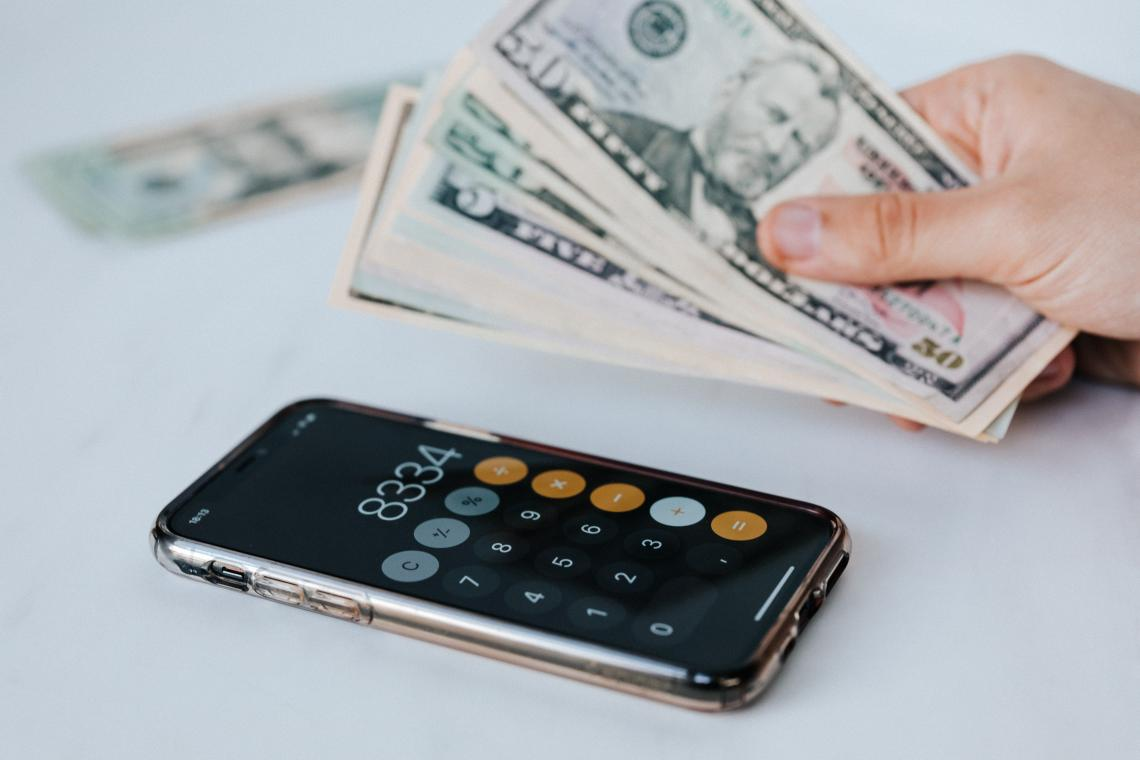FL estimates how much a business borrows to grow its assets and net income. This way, we can evaluate changes in earnings before interest and taxes concerning earnings per share.

How do we calculate the Degree of FL?

Degree of FL = Change in Earnings before interest and taxes/ Change in Earnings before taxes.

Or

Degree of FL = Percentage change in Net Income/ Percentage change in Operating Income

What is Financial Risk?

It is the risk related to a company's method of financing its operational/business activities.

If a business uses debt to support its operations, it is under the legal obligation to repay the sums that make up its debts when they are due.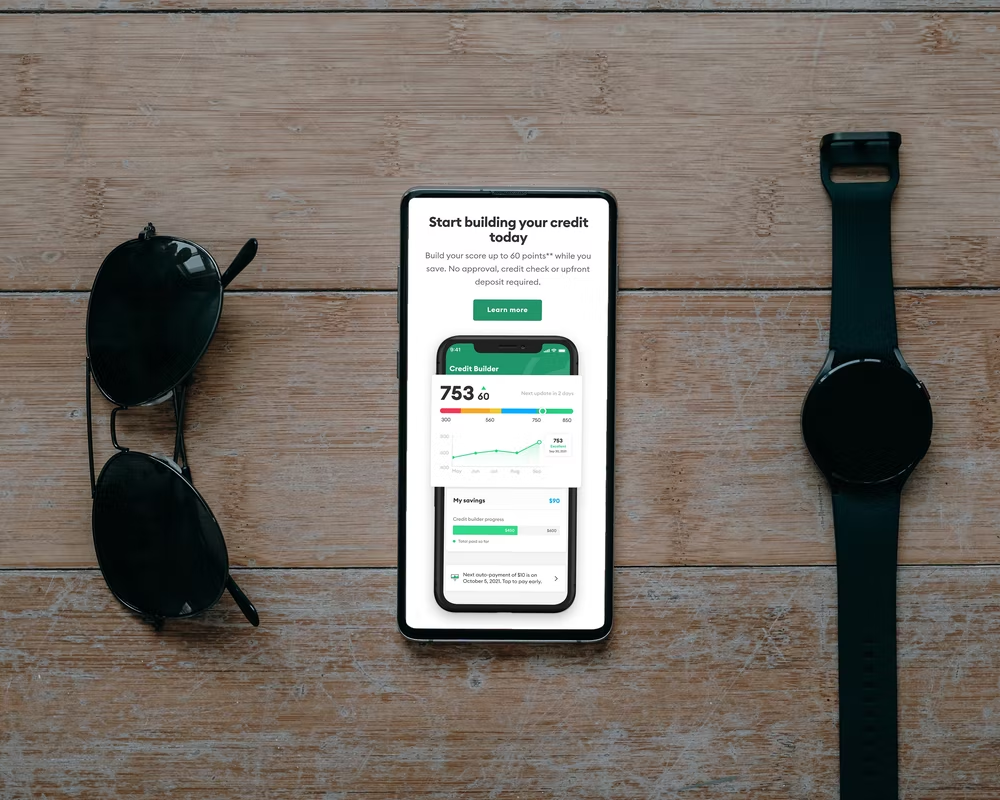The firm raises its financial risk by taking on fixed obligations like debt and long-term leases. However, a firm does not have set commitments if it finances its activities using common stock (equity) through operations (retained earnings) or by issuing additional common shares

Hence higher the fixed cost obligations, i.e., debt, the higher the risk associated with that firm.

## Degree of Total Leverage (TL)

Together, these two components - degree of financial and operating leverage- form the degree of total leverage in a business.Now, let us understand the degree of TL in simple mathematical terms. We can define it as follows:

Degree of TL = Degree of OL* Degree of FL

Or

Degree of TL = (Percentage change in Net Income)/ (Percentage change in the number of units sold)

The fixed operational costs serve as a pivot in the case of OL. As a result, operating income is more susceptible to variations in sales as the higher the constant share of operating expensesThe fixed financial costs, such as interest, serve as a pivot in the case of FL. The more operating income is financed through sources with fixed costs, such as debt, the more volatile the cash flows accessible to shareholders.

Generally, we can observe that fixed operating and finance costs raise the sensitivity of earnings to owners by combining the effects of both types.

Earlier, we mentioned breakeven points. So let us look at breakeven points and operating breakeven points.

The number of units produced and sold at which the business experiences a net loss or the point at which revenues and costs are equal is known as the breakeven point.

When revenues and total expenditures are plotted against the number of units manufactured and sold, the intersection point is the number of teams we need to break even. When these many units are manufactured and sold, revenues and costs are equal; the profit is zero.

Here's how the breakeven point is calculated mathematically:

PQ = VQ + F + C

Where:

P = the price per unit

Q = the number of units produced and sold

V = the variable cost per unit

F = the fixed operating costs

C = the fixed financial cost

Therefore,

PQ(BE) = VQ(BE) + F + C

Which gives the breakeven number of units, Q(BE), as

Q(BE) = (F + C)/ (P - V)

Where BE stands for Breakeven point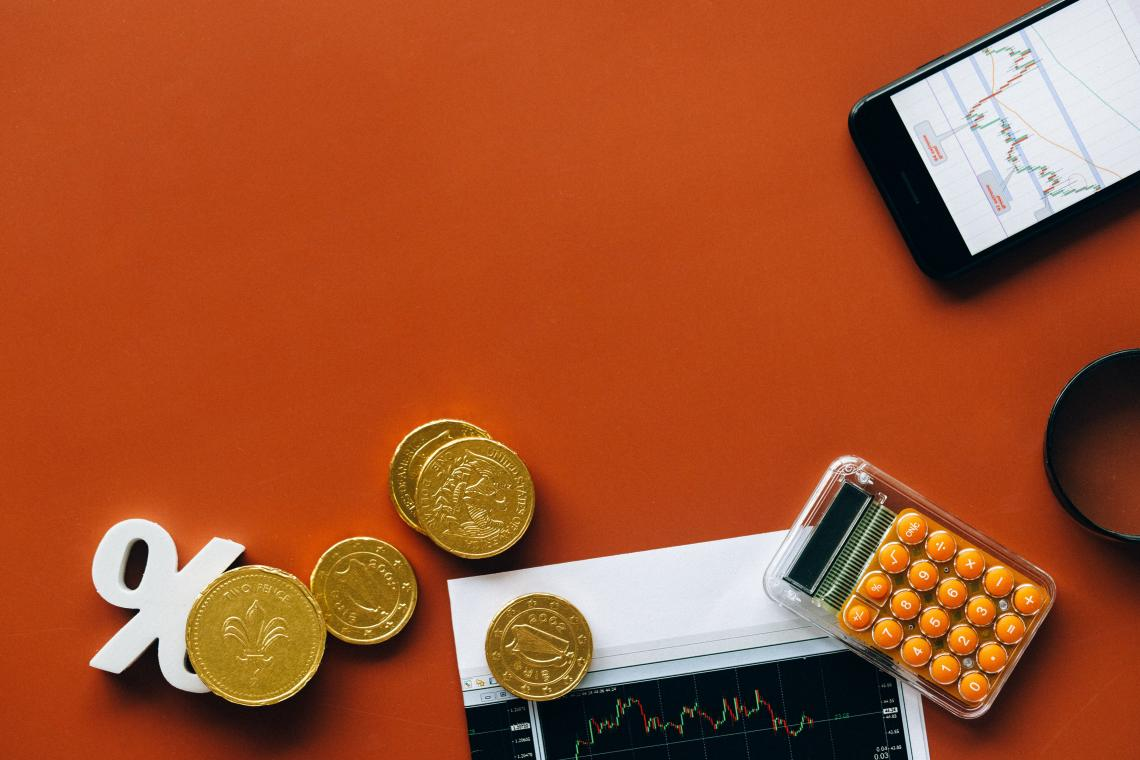We can also indicate the breakeven point in terms of operating profit, which we refer to as the operating breakeven point, or Q(OBE), in addition to the breakeven point provided in terms of net income or Q(BE).

The operational breakeven number of units, or Q(OBE), is calculated by setting revenues at the operating breakeven point equal to operating costs at the operating breakeven point.

The calculation demonstrates that Q(OBE) is equal to the difference between the price per unit and variable cost per unit divided by fixed operating costs:

PQ(OBE) = VQ(OBE) + F

Q(OBE) = F/(P - V)

Where,

OBE stands for Operating breakeven point.### Everything You Need To Build Your Accounting Skills

To Help You Thrive in the Most Flexible Job in the World.

Researched and Authored by Punit Manjani | LinkedIn